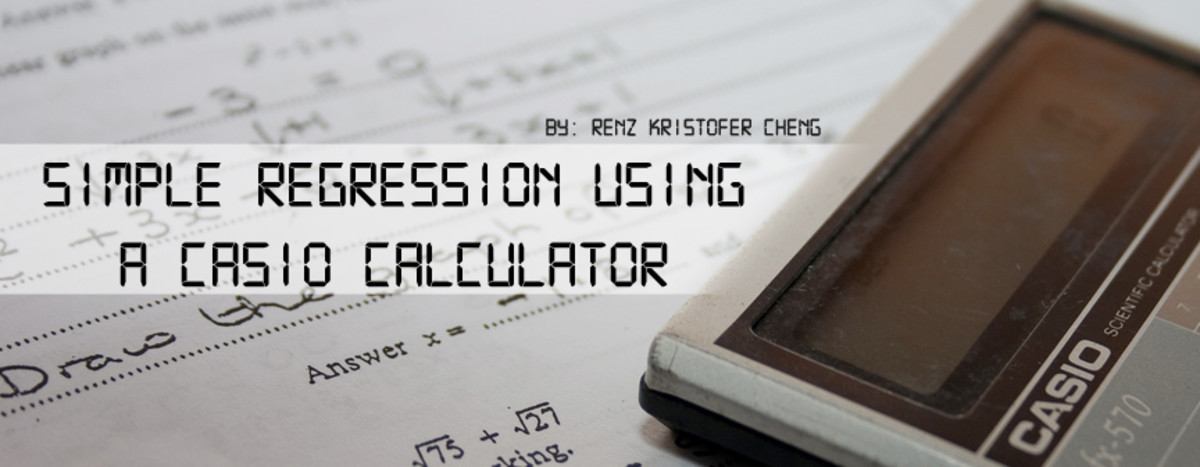# How to Do a Simple Regression using a Casio CalculatorDoing regression analysis using a CASIO calculator_M-j-H_, CC BY, via flickr

## Statistics and Simple Regression

Statistics may be something that a lot of students dread (and even those who are now working). All those complicated computations simply make you go crazy! Take for example regression analysis. It requires several computations to arrive with values for the intercept, slope and etc. And doing it manually, which is something needed in class, can be a real source of headache. One mistake would make everything go wrong.

So, is there a way for us to verify our answers and even aid us in our calculations? Well, fortunately there is! You just need a calculator that has this special function. In this article, we will know how to utilize that function using a CASIO calculator.

XY

240

3.6

236

3.4

290

4.3

500

8.1

630

10.2

444

7.1

## Learning by Doing

To help you out in learning how to do it, let's use the table on the right as an example. We will be conducting a simple regression using these values.

Okay, so now, grab your calculator and let's get going!

## Simple Regression Using Casio Calculator

So, here are the 6 basic steps on how you can conduct a simple regression in your calculator:

1. First, press on the MODE button to make 8 mode options appear in your display. Then, among those options, press the number that corresponds to STAT.
2. Again, there will be another set of 8 options to choose from. Press the number that corresponds to A+BX since this is the function for conducting a simple linear regression.
3. Input all the data (see the previous table), and press AC afterwards.
4. Press the SHIFT button followed by the STAT button (this is usually the button corresponding to 1). Then, press on the number indicating REG (which is typically 7).
5. Okay, we are getting nearer! Now, to solve for the intercept (represented by "A"), press 1; for the slope (represented by "B"), press 2; and for R (represented by "r") , press 3 followed by the "=" sign. Again, numbers to press may vary depending upon the model of your calculator.
6. Repeat Step 5 for the other requirements (e.g. slope, R, etc)
Scroll to Continue

To check if you were able to do it right, here are the answers you should be getting (rounded off in 5 decimal places):

a (intercept) = -0.63035

b (coefficient) = 0.01730

R = 0.99961

If you were able to get them all, then congratulations. If not, you may want to check the step-by-step procedures, and make sure you are not missing anything. Check also if you were able to input the data correctly, since it's usually the common error.

## Important Note

It is essential to know that not all CASIO scientific calculators have this function. Also, the steps enumerated above may not be applicable to other brand of calculators. If in case you'll ask, SHARP also have calculators that can do simple regression analysis.

## Mission Accomplished

Hopefully, you found this guide simple yet useful. Now, you have a better chance to get higher scores in your statistics exams. Leave feedback if you are encountering problems.

Also, you may want to learn how to solve for x using your calculator. Just check out the article by clicking on the link.